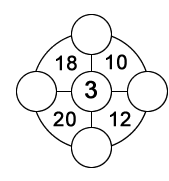Select PagePrintable version

The number within the four sectors of the outer circle is equal to the sum of the three numbers next to its sector.  The numbers in the individual circles can only be 1 to 9 and each number can be used only once. One number has been provided to get you started.  Find the remaining four numbers.### Solution### Solution explanationComments on solving the puzzle; a process of eliminating possible values as answers:

Value 3 is provided and can not be reused.

Starting at Equation (1), unknowns A & B can only be equal to 1, 2, 5 or 6 as shown. All other combination include a 3 value.

Equation (2): unknown B can now be equal to 1, 2 or 5 only as shown Then C can only be equal to 4, 7, or 8. Comparing with Equation (1), 6 is eliminated as a possible value for B, A can now only be equal to 2, 5 or 6.

Equation (3): unknowns C & D can only be equal 8 or 9 as shown. Therefore comparing with Equation (2), C must be equal to 8 & D equal to 9. With C equal to 8 in Equation (2), B must then be equal to 1 and with B equal to 1 in Equation (1), A must then be equal to 6.

Equation (4) confirms that if D is equal to 9 and A is equal to 6.

Now insert your final answers into the original puzzle and verify the resulting sums.